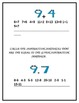# Common Core Math Review for Grade One: Operations and Algebraic ThinkingResource Type
File Type
Word Document File (291 KB|22 pages)
Standards
\$5.00
• Product Description
• Standards
This Common Core Math Review for Grade One: Operations and Algebraic Thinking will provide all first-grade teachers with a quick, effective assessment of their first-grade students' math skills according to the common core standards. Solving word problems, through addition and subtraction, whose sums and/or differences are within 20, identifying number sentences that are true or false within the sum or difference of 20 through the understanding of the commutative property concept, creating number sentences whose sum or difference is within 20, determining unknown whole numbers to make true addition and subtraction equations, and solving addition word problems that contain three whole numbers, whose sum is up to 20, by counting the pictured objects which accompany each given story problem are just some of the skills covered in this math unit on Operations and Algebraic Thinking. In addition, students will have an opportunity to draw objects to represent given math equations as well as to express their understanding of the meaning of the equal sign. An effective assessment to use on any day of the week!

This product has been used in real classrooms with real students with real success! It is created by two experienced teachers with over 24 years of combined teaching experience mixed with current essential knowledge that has been acquired through teacher inservices, professional development courses, educational conferences, peer collaborations, and just everyday on-the-job experiences with our students, our parents, and our peers!
Thanks so much!!! :) The Teachers’ Corner
Graphics provided by www.mycutegraphics.com
Determine the unknown whole number in an addition or subtraction equation relating three whole numbers. For example, determine the unknown number that makes the equation true in each of the equations 8 + ? = 11, 5 = ▯ - 3, 6 + 6 = ▯.
Understand the meaning of the equal sign, and determine if equations involving addition and subtraction are true or false. For example, which of the following equations are true and which are false? 6 = 6, 7 = 8 - 1, 5 + 2 = 2 + 5, 4 + 1 = 5 + 2.
Add and subtract within 20, demonstrating fluency for addition and subtraction within 10. Use strategies such as counting on; making ten (e.g., 8 + 6 = 8 + 2 + 4 = 10 + 4 = 14); decomposing a number leading to a ten (e.g., 13 - 4 = 13 - 3 - 1 = 10 - 1 = 9); using the relationship between addition and subtraction (e.g., knowing that 8 + 4 = 12, one knows 12 - 8 = 4); and creating equivalent but easier or known sums (e.g., adding 6 + 7 by creating the known equivalent 6 + 6 + 1 = 12 + 1 = 13).
Relate counting to addition and subtraction (e.g., by counting on 2 to add 2).
Understand subtraction as an unknown-addend problem. For example, subtract 10 – 8 by finding the number that makes 10 when added to 8.
Total Pages
22 pages